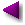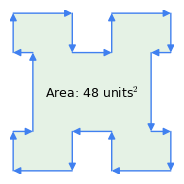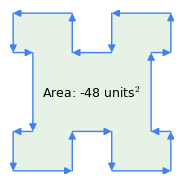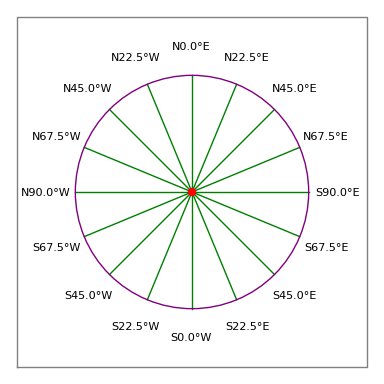Home | Mathematics | * Consumer Loan Calculator * Applied Mathematics * Calculus * Finance Calculator * Is Mathematics a Science? * Maxima * Sage * Trigonometric Relations * Unit Conversions Area of an Irregular Polygon Binomial Probability Equities Myths Graphinity Graphitude Interactive LaTeX Editor Introduction to Statistics Mandelbrot Set Peak People Polygon Calculator Polynomial Regression Data Fit Polynomial Regression Data Fit (Java version) Prime Numbers Quadratic Equation Solver Randomness Signal Processing The Mathematics of Pi The Mathematics of Population Increase The Physics Behind Stopping a Car Why PDF?Share This Page
Area of an Irregular Polygon
An easy solution to a difficult problem
Version 1.3 (04.15.2013)
(double-click any word to see its definition)
Note: In this article, footnotes are marked with a light bulb over which one hovers.
Introduction

This article describes a simple solution to a geometric problem, one that I find described in overly complex ways online. The problem is to compute the area and perimeter length of a two-dimensional, closed figure like a room's floor plan, or a plot of land, or any other two-dimensional bounded figure (an "irregular polygon"), regardless of how complex.

I certainly don't claim originality for this method. Versions have existed since the time of Gauss or before. But most online articles describe the method in unnecessarily complex ways. References include the Wikipedia Shoelace Formula article, a similarly complex method in the Polygon article, and a number of articles that seem calculated more to demonstrate the author's erudition than to provide the least complex embodiment of the method. This article (PDF) shows one of the least complex (but by no means simple) expressions:

(1) $A = \frac{1}{2} \left\{ \left|\begin{array}{cc} x_0 & x_1 \\ y_0 & y_1\\ \end{array} \right| + \left|\begin{array}{cc} x_1 & x_2 \\ y_1 & y_2\\ \end{array} \right| + \left|\begin{array}{cc} x_2 & x_3 \\ y_2 & y_3\\ \end{array} \right| + ... + \left|\begin{array}{cc} x_{n-2} & x_{n-1} \\ y_{n-2} & y_{n-1}\\ \end{array} \right| + \left|\begin{array}{cc} x_{n-1} & x_0 \\ y_{n-1} & y_0\\ \end{array} \right| \right\}$

Where $x_n,y_n$ is a polygon vertex expressed in Cartesian coordinates, and $\left|\begin{array}{cc} x_0 & x_1 \\ y_0 & y_1\\ \end{array} \right|$ refers to a determinant, with the value $x_0 \times y_1 - y_0 \times x_1$

Don't be discouraged by the above exposition — using this method is easier than understanding its mathematical underpinnings. While researching this article I found any number of replies to student inquiries that were so complex that they couldn't possibly have enlightened the student. In this article I'm going to do all I can to make this method as simple and accessible as possible.

Computing Area and Perimeter
The method is most easily expressed as an algorithm:
• An irregular polygon's vertices can be represented as Cartesian coordinates: $x,y$.
• Each pair of coordinates is processed in an area summation algorithm that looks like this:
$A = A + \frac{(x_1 \times y_0 - y_1 \times x_0)}{2}$
$A = A + \frac{(x_2 \times y_1 - y_2 \times x_1)}{2}$
...
• A simple mathematical expression for the above would be:
(2) Irregular polygon area $\displaystyle A = \frac{1}{2} \sum_{i=m}^{n-1}{x_{i+1} \times y_i - y_{i+1} \times x_i}$
• NOTE: In equation (2), the traversed figure ends with the last provided data item, it doesn't repeat the initial item as shown in equation (1) above. For my purposes, explained below, this form is intentional — in a land survey the field data should close the figure, i.e. the final item should equal the first. In such an application, to automatically close the figure as in equation (1) would conceal errors that need to be evaluated.

Users may prefer the form shown in equation (1) above, for purposes other than land survey data reduction. For those purposes, during processing simply reëvaluate the first data item at the end of the traversal, or provide data sets that repeat the first data item as the last.

• Here is a complete, working Python function:
def find_area(array):
a = 0
ox,oy = array
for x,y in array[1:]:
a += (x*oy-y*ox)
ox,oy = x,y
return a/2

• The "array" argument above is a set of Cartesian $x,y$ coordinates, i.e. ((10,10),(10,20),(20,20),(20,10),(10,10)). Notice about this data set that the first data item is repeated at the end.

• Here is a slightly more complex Python function that computes both area and perimeter length:
def find_area_perim(array):
a = 0
p = 0
ox,oy = array
for x,y in array[1:]:
a += (x*oy-y*ox)
p += abs((x-ox)+(y-oy)*1j)
ox,oy = x,y
return a/2,p

• Again, this reminder: for both the above code examples, in applications other than land survey data reduction, the provided data set should include a copy of the first data item as the last, i.e. the figure should be explicitly closed.

• I chose Python for these examples because a Python listing maximizes algorithmic meaning while minimizing cruft.

• The above functions can easily be converted into other languages, almost directly — except the perimeter summation shown above, which exploits the fact that Python supports complex numbers. For languages that don't support complex numbers, one may create the perimeter result this way:

C/C++: p += sqrt(pow(x-ox,2)+pow(y-oy,2));

Java: p += Math.sqrt(Math.pow(x-ox,2)+Math.pow(y-oy,2));

• Formally, the perimeter length is the sum of the vector magnitudes:
(3) Perimeter length $\displaystyle P = \sum_{i=m}^{n-1}{\sqrt{(x_{i+1}-x_i)^2 + (y_{i+1}-y_i)^2}}$Figure 1: Clockwise vs. counterclockwise vectors

Here are some important properties of this method:

• The origin vertex chosen within the polygon is irrelevant to either area or perimeter calculation, only that the figure be closed, i.e. the last coordinate should equal the first.
• "Clockwise Rule": the order of the vectors is significant — if the perimeter is traversed clockwise, the area result is positive, if counterclockwise, it's negative (Figure 1). It's this property of the method that subtracts the area of inflections from a complex figure as in Figure 1 — the "inflections" represent a counterclockwise traversal within the polygon.
• Many applications of this method use original data sources consisting of polar vectors (an example is land survey legal descriptions), but this method requires Cartesian coordinates. The conversion from polar to Cartesian coordinates is trivial, but it's important to keep this requirement in mind.
• Again, for an accurate area calculation, the described polygon should be a closed figure — the last coordinate should equal the first. For applications using field data consisting of length vectors that are subsequently converted to Cartesian form, it's a good idea to compare the beginning and ending Cartesian coordinates — they should be equal. For example, older land surveys (before the era of cheap computer power) regularly fail this test.
Area/Perimeter Computer

This section is a fully functional area/perimeter computer that can process entered Cartesian or polar coordinate data pairs, or survey legal descriptions. Just choose a data type (cartesian x,y coordinates, polar m,θ coordinates or a survey legal description) and enter (or paste) data into the data entry area below. You may also select sample data sets for familiarization and practice.

Because of browser security issues, to avoid excessive typing and to import significant amounts of data from another source, readers will need to use their system clipboard. Just copy data onto the clipboard at the source application, then click the data entry area below and press Crtl+A to select all, Ctrl+V to pase data from elsewhere, and Ctrl+C to copy your own entered data. Most browsers include clipboard functions as menu items as well.

 Choose data type: Cartesian (x,y) polar (m,θ) Survey description

Data Entry Area: Select All: Ctrl+A | Copy: Ctrl+C | Paste: Ctrl+V

 

Chart Area:

Sorry — your browser doesn't support the graphic ability this feature requires —
consider installing Google Chrome or Firefox.

 Options: Lines Arrows Origin Center Length Unit: Foot Inch Meter Centimeter Line width: 1 2 3 4 5 Reverse

Results:Select All: Ctrl+A | Copy: Ctrl+C | Paste: Ctrl+V

Input Data Types

The area/perimeter computer has three input modes:

• Cartesian (x,y) coordinate pairs:

• Each coordinate is a two-dimensional position with a horizontal (x) and vertical (y) part (a Cartesian coordinate).
• The coordinates aren't cumulative — each independently describes its location.
• So a square would be:
• 0,0 (origin)
• 0,100 (up)
• 100,100 (right)
• 100,0 (down)
• 0,0 (back to the origin)
•Figure 2: Compass rose
• The commas in the above example are only meant to group the data pairs for the reader — they have no significance to the area computer's data parser.
• The parser is robust enough that the above example can be pasted into the computer's data entry area and it will work (try it).
• The only limitation is that explanatory comments can't contain numbers.

• Polar (m,θ) coordinate pairs:

• Each coordinate consists of a distance and an angle in degrees (a polar vector).
• The angles follow the compass convention — 0 degrees is up or "north", 90 degrees is to the right or "east" (Figure 2).
• Unlike the prior Cartesian form, these coordinates are cumulative — each new vector adds to the present position.
• So a square would be:
• 100,0 (up)
• 100,90 (right)
• 100,180 (down)
• 100,270 (back to the origin)
• As before, this example will work if pasted into the computer's data entry area and the polar mode selected.
• Notice about polar coordinates that the origin is implicit and preset at x = 0, y = 0.Figure 3: Surveyor's compass rose

• Legal description:

• First, an explanation of the relationship between the "Length Units" selection and the results display:
• For each polygon, a list of area results is computed using a fixed set of common area units (feet2, meters2, etc.). For this area list to be meaningful, the input length units must be known.
• In Cartesian and polar mode, data entries have no units, so the user chooses what units to assign to lengths. So when the user changes length units, the area values change, the length labels change, but the length values don't.
• In Survey mode, the legal description contains length units (feet, meters, etc.) which means the length units are predefined by the source. So when the user changes length units, this changes length labels and values, not area values.
• The survey description parser is by far the least reliable of the three data parsing methods — it's more of an experiment than a robust data conversion method.
• I wrote this parser by downloading a number of legal descriptions (which are provided as examples in the above calculator) and tuning the parser's code until all could be successfully decoded.
• Criteria for "success" were that the result would have the same square area as the description claims, and that the figure "close".
• The term "closure", and particularly error of closure, is used in survey work to describe the degree to which the end point of the survey traverse is equal to the beginning point. This is rarely true in reality — errors in closure usually arise from errors in field work, and older surveys, published before the advent of cheap computer power, tend to have rather poor closures as well as wildly inaccurate estimates of square area.
• A survey legal description is a formal way to describe a plot of land — its form and syntax has arisen over decades by mutually accepted, somewhat arbitrary, conventions shared by surveyors.
• For this area/perimeter computer the entered data must follow certain strict conventions that some, but not all, legal descriptions adhere to. The essential elements are:
• The existence of the phrase "point of beginning", that separates some preliminary content from that describing the dimensions of the parcel being described. Some documents say "True point of beginning", some say "Real point of beginning" and some say "Point of beginning". A successful parser needs to remove the content before this phrase and retain all after it.
• A series of phrases that describe a special kind of polar vector used by surveyors:
• The word "North" or "South", or its abbreviation "N" or "S".
• Then an angle expressed in degrees, minutes and seconds.
• Then the word "East" or "West", or its abbreviation "E" or "W".
• Then a distance.
• Then a distance unit — feet, meters, etc.
• Here are some different examples of surveyor vectors from the sample legal descrtiptions:
• N 2°0'0" W — 63.50 feet
• N90°00’00”W ALONG A LINE PARALLEL WITH SAID NORTH LINE A DISTANCE OF 6.00 FEET
• North 0°06'O0" West a distance of 554.86 feet
• S. 22 degrees 41′ 55″ E. 174.10 feet
• Notice about the above examples that they all have different syntax. There are many legal descriptions in existence that this parser won't successfully decode, only because I haven't tried to incorporate their syntax. But it's important to add that a truly robust parser for the multitude of legal descriptions in existence would have to be as smart as Watson.
• Users of this area/perimeter computer may want to avoid submitting plain-text legal descriptions and hoping the parser will figure it out. To make direct hand entries (or to create a list of traverses elsewhere for importation), use this convention:
• Remember that an acceptable traverse entry consists of the word "North" or "South", or the letter "N" or "S", followed by up to three numbers providing degrees, minutes and seconds of angle, followed by the word "East" or "West" or the letter "E" or "W", followed by a distance, followed by a distance unit.
• So an acceptable entry would be "N 30 15 45 W 100 feet", meaning a traverse of 100 feet pointing 30 degrees, 15 minutes and 45 seconds West of North (i.e. roughly 330 compass degrees, see Figure 3).
• The minutes and seconds entries may be skipped if they are equal to zero, or if the entry is in decimal degrees, or degrees and decimal minutes.
• It's not difficult to convert a printed legal description into such entries, and there are a number of ways to detect errors. After all the traverses have been entered, if the figure has no closure error and reports the same square area as the original, chances are the entries are correct (or they have the same errors as the original description).
• After entering a legal description this way, if there is an unacceptable closure error or the square area is wrong, remember that the original data might be wrong. There are any number of legal descriptions in existence that have never been checked against a computer method like this, and that were never correct.

Notes

While browsing the Cartesian (x,y) examples, notice the different shapes' ratio of area to perimeter. The "oddly shaped polygon" has an intermediate ratio, the circle has the smallest ratio, and the Hilbert space has by far the largest ratio. This relationship extends into higher dimensions, where a sphere is known to have the greatest volume per surface area.

About the circle example — it's a "unit circle", a circle with a radius of one. For the provided example, the area approximates $\pi$ and the perimeter approximates $2\pi$, the right values for a unit circle. For those values to be more than approximations, to be exactly equal $\pi$ and $2\pi$, the number of polygon sides would have to be infinite. I decided against this. :)

The Hilbert space example was generated using a Sage algorithm with a modest order choice (to avoid generating too much data). In principle a Hilbert space can have an infinite ratio of perimeter to area in two dimensions, or surface area to volume in three dimensions. To see how complex a Hilbert space can become, click here to see an order-7 Hilbert space.

Notice about the Hilbert example that the computed area is close to 1/2 for a 1x1 surface. Ideally, without the necessity to convert a line figure into an area figure and with the right algorithm, the surface area would be exactly 1/2 that of a square of the same dimensions.

The polar entry mode is more or less equal to Turtle graphics, if one substitutes zero degrees for "up", 90 degrees for "right", etc., and if each turn and move sequence is collapsed into one command. Using the polar entry mode is an easy way to hand-construct a complex shape, because distances and angles are easier to keep track of than Cartesian coordinates.

I first used the above-described land survey area computing method years ago when I lived in rural Oregon, surrounded by people who needed to know the area and perimeter of their properties. I encountered many shocking examples where real estate agents brazenly cheated people who had no way to find out what was going on. On any number of occasions I would compute an area from a legal description, only to discover that the warranty deed's claim about acreage was wildly inaccurate — and always higher than reality.

On one occasion I was asked to split a property into two equal sections and create legal descriptions for each. Because I had a newly acquired Apple II (which had the processing power of a modern pencil sharpener), this was very easy — but as I worked I realized the total acreage was off by about three acres, about 25% of the total. I confronted the title company, which brazenly replied, "See here, where the deed says 'more or less'?"

On another occasion I began a land purchase, but forewarned by the above experience, when I received the title policy I decided to enter its legal description into my program to see if the acreage claim was accurate. But to my horror, I discovered that all the title policies for the parcels in that section had the same legal description — of the first surveyed property. It turns out that the land development company decided to save money by surveying just one of the dozens of lots offered for sale, and include that legal description in all the title policies. They were sure they would get away with it — after all, who knows anything about mathematics?

After arguing with the title company and the developer, trying to persuade them to pay for surveys so the legal descriptions weren't polite fictions, I gave up and decided not to buy the land. It was like finding out how sausage is made — it's possible to know too much.

Version History
• 04.15.2013 Version 1.3. Added a detailed explanation of the default data format, i.e. lists that repeat the first data point as the last.
• 03.24.2013 Version 1.2. Further optimized the survey description parser, added more result data fields.
• 03.23.2013 Version 1.1. Improved the legal description parser to deal with more kinds of descriptions, added length units selector and a result list of areas in common units.
• 03.22.2013 Version 1.0. Initial Public Release.

 Home | Mathematics | * Consumer Loan Calculator * Applied Mathematics * Calculus * Finance Calculator * Is Mathematics a Science? * Maxima * Sage * Trigonometric Relations * Unit Conversions Area of an Irregular Polygon Binomial Probability Equities Myths Graphinity Graphitude Interactive LaTeX Editor Introduction to Statistics Mandelbrot Set Peak People Polygon Calculator Polynomial Regression Data Fit Polynomial Regression Data Fit (Java version) Prime Numbers Quadratic Equation Solver Randomness Signal Processing The Mathematics of Pi The Mathematics of Population Increase The Physics Behind Stopping a Car Why PDF?Share This Page